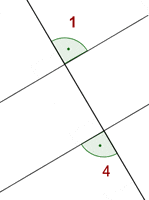Learn from home

The teachersAn angle is the region of a plane between two rays that share a common origin. The rays are called arms and the common origin is the vertex.To measure angles, the unit degree (º) is most frequently used.

## Acute AngleAn acute angle measures between and 90º.

## Right AngleA right angle measures exactly 90º.

## Obtuse AngleAn obtuse angle measures between 90º and 180º.

## Straight AngleA straight angle measures exactly 180º.

## Reflex AngleAn reflex angle measures between 180º and 360º.

## Negative AngleA negative angle measures less than 0º.

Negative angles rotate in a clockwise direction.

A negative angle can be transformed into a positive angle by adding 360º to it.

−30º = 360º − 30º = 330º

## Congruent AnglesCongruent angles have the same measure.Adjacent angles have a common side and a common vertex.

## Complementary AnglesComplementary angles sum 90°.

## Supplementary AnglesSupplementary angles sum 180°.

## Vertical Angles or Opposite AnglesThe vertical angles share the common vertex and the sides of one are an extension of the sides of the other.

The angles 1 and 3 are equal.

The angles 2 and 4 are equal.

## Corresponding AnglesThe angles 1 and 2 are equal.

## Alternate Interior AnglesThe angles 2 and 3 are equal.

## Alternate Exterior AnglesThe angles 1 and 4 are equal.

## Operations with Angles

The sum of two angles is another angle whose amplitude is the sum of the amplitudes of the two initial angles.### Subtracting Angles

The subtraction of two angles is another angle whose amplitude is the difference between the amplitude of the higher angle and the lower angle.### Multiplying an Angle by a Number

Multiplying a number by an angle is another angle whose amplitude is the amplitude of the angle multiplied by as many times as specified by the number.### Dividing an Angle by a Number

The division of an angle by a number is another angle that is multiplied by this number and gives the original angle.:4 =Did you like the article?(1 votes, average: 5.00 out of 5)Loading...

Emma

I am passionate about travelling and currently live and work in Paris. I like to spend my time reading, gardening, running, learning languages and exploring new places.

Did you like
this resource?

Bravo!